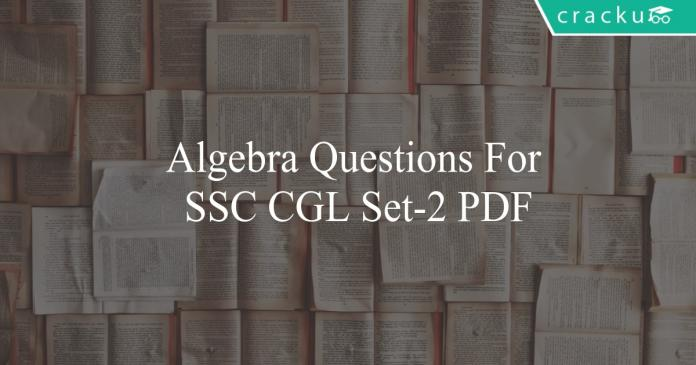# Algebra Questions For SSC CGL Set-2 PDF

0
1448algebra questions for ssc cgl set-2 pdf

# Algebra Questions For SSC CGL Set-2 PDF

Download SSC CGL Algebra questions with answers PDF based on previous papers very useful for SSC CGL exams. 20 Very important Algebra objective questions (MCQ’s) for SSC exams.

Question 1: If If $(3^{x})(3^y) = 9$ and $(5^{x})(125^y) = 625$, then find (x,y)

a) (4,-2)

b) (0,2)

c) (1,1)

d) (6,-4)

Question 2: The lines 2x+y = 3 and x+2y = 3 intersect at points

a) (1,1)

b) (-1,5)

c) (0,3)

d) (3,-3)

Question 3: Find the value of $\Large\frac{\frac{1}{2}.\frac{1}{2}.\frac{1}{2}+\frac{1}{4}.\frac{1}{4}.\frac{1}{4}+\frac{1}{5}.\frac{1}{5}.\frac{1}{5}-3.\frac{1}{2}.\frac{1}{4}.\frac{1}{5}}{\frac{1}{2}.\frac{1}{2}+\frac{1}{4}.\frac{1}{4}+\frac{1}{5}.\frac{1}{5}-(\frac{1}{2}.\frac{1}{4}+\frac{1}{4}.\frac{1}{5}+\frac{1}{2}.\frac{1}{5})}$

a) $\large\frac{17}{20}$

b) $\large\frac{19}{20}$

c) $\large\frac{13}{20}$

d) $\large\frac{11}{20}$

Question 4: Find the value of $\Large\frac{\frac{1}{3}.\frac{1}{3}.\frac{1}{3}+\frac{1}{4}.\frac{1}{4}.\frac{1}{4}+\frac{1}{9}.\frac{1}{9}.\frac{1}{9}-3.\frac{1}{3}.\frac{1}{4}.\frac{1}{9}}{\frac{1}{3}.\frac{1}{3}+\frac{1}{4}.\frac{1}{4}+\frac{1}{9}.\frac{1}{9}-(\frac{1}{3}.\frac{1}{4}+\frac{1}{4}.\frac{1}{9}+\frac{1}{3}.\frac{1}{9})}$

a) $\large\frac{25}{36}$

b) $\large\frac{19}{36}$

c) $\large\frac{24}{35}$

d) $\large\frac{17}{26}$

Question 5: What is the square root of 97-16$\sqrt{3}$

a) 9-4$\sqrt{3}$

b) 9+4$\sqrt{3}$

c) 7-4$\sqrt{3}$

d) 7+4$\sqrt{3}$

Question 6: Find the number of prime factors of 14560

a) 3

b) 4

c) 5

d) 6

Question 7: Find the number of even factors of 15680.

a) 42

b) 21

c) 36

d) 24

Question 8: Find the number of even factors of 16200.

a) 60

b) 42

c) 36

d) 45

Question 9: Find the number of even factors of 3920.

a) 24

b) 42

c) 36

d) 30

Question 10: Find the number of odd factors of 13500.

a) 24

b) 32

c) 16

d) 48

Question 11: Find the number of prime factors of 5544.

a) 7

b) 4

c) 6

d) 3

Question 12: What is the square root of $214 – 78\sqrt{5}$

a) $13-3\sqrt{5}$

b) $17-4\sqrt{5}$

c) $13-6\sqrt{5}$

d) $17-3\sqrt{5}$

Question 13: If $(9^{x})(3^y) = 81$ and $(2^{x})(4^y) = 32$, then find $(x, y)$

a) (1,4)

b) (2,1)

c) (1,2)

d) (4,1)

Question 14: If p = 21, q = -16, r = -5, then find the value of $\large\frac{8p^{3}+8q^{3}+8r^{3}}{9pqr}$

a) $\Large\frac{8}{3}$

b) $\Large\frac{16}{3}$

c) $\Large\frac{8}{9}$

d) $\Large\frac{8}{16}$

Question 15: Find the value of $\sqrt{12+\sqrt{12+\sqrt{12+……}}}$

a) 4

b) 3

c) 6

d) 7

Question 16: What is the units digit of $437^{79}$ ?

a) 7

b) 9

c) 1

d) 3

Question 17: If $(p-q)^{2} = 9$ and $(p+q)^{2} = 121$ $p,q>0$, then find the value of $\frac{pq}{p+q}$

a) $\frac{22}{13}$

b) $\frac{24}{13}$

c) $\frac{24}{11}$

d) $\frac{28}{11}$

Question 18: What is the units digit of $639^{147}$ ?

a) 7

b) 9

c) 1

d) 3

Question 19: If $3p – (1/2)(p -3) = 14$, find p ?

a) 2

b) 3

c) 4

d) 5

Question 20: A fraction is greater than its reciprocal by $\Large\frac{9}{20}$. What is the fraction?

a) $\Large\frac{5}{4}$

b) $\Large\frac{-4}{5}$

c) $\Large\frac{1}{5}$

d) Either A or B.

Answers & Solutions:

1) Answer (C)

Given $(3^{x})(3^y) = 9$

=> $3^{x+y} = 3^{2}$

=> x+y = 2 — (1)

$(5^{x})(125^y) = 625$
=> $(5^{x})((5^3)^y) = 5^4$
=> $(5^x)(5^3y) =5^4$
=> $5^x+3y = 5^4$
=> $x+3y = 4$ — (2)
Solving (1) and (2)

=> 2y = 2 => y = 1
Substituting y = 1 in (1) –> x = 1

Therefore, (x,y) = (1,1)

2) Answer (A)

Given 2x+y = 3 and x+2y = 3
Solving above equations,
We get x = 1 and y = 1.
Hence, the lines intersect at (1,1)

3) Answer (B)

The given equation is in the form of
$\large\frac{a^3+b^3+c^3-3abc}{a^2+b^2+c^2-(ab+bc+ca)}$

We know that $a^3+b^3+c^3-3abc = (a+b+c)(a^2+b^2+c^2-(ab+bc+ca))$

=> $\frac{a^3+b^3+c^3-3abc}{a^2+b^2+c^2-(ab+bc+ca)} = a+b+c$

Then, $\Large\frac{\frac{1}{2}.\frac{1}{2}.\frac{1}{2}+\frac{1}{4}.\frac{1}{4}.\frac{1}{4}+\frac{1}{5}.\frac{1}{5}.\frac{1}{5}-3.\frac{1}{2}.\frac{1}{4}.\frac{1}{5}}{\frac{1}{2}.\frac{1}{2}+\frac{1}{4}.\frac{1}{4}+\frac{1}{5}.\frac{1}{5}-(\frac{1}{2}.\frac{1}{4}+\frac{1}{4}.\frac{1}{5}+\frac{1}{2}.\frac{1}{5})}$ $= \large\frac{1}{2}+\frac{1}{4}+\frac{1}{5} = \frac{10+5+4}{20} = \frac{19}{20}$

4) Answer (A)

The given equation is in the form of
$\large\frac{a^3+b^3+c^3-3abc}{a^2+b^2+c^2-(ab+bc+ca)}$

We know that $a^3+b^3+c^3-3abc = (a+b+c)(a^2+b^2+c^2-(ab+bc+ca))$

=> $\frac{a^3+b^3+c^3-3abc}{a^2+b^2+c^2-(ab+bc+ca)} = a+b+c$

Then, $\Large\frac{\frac{1}{3}.\frac{1}{3}.\frac{1}{3}+\frac{1}{4}.\frac{1}{4}.\frac{1}{4}+\frac{1}{9}.\frac{1}{9}.\frac{1}{9}-3.\frac{1}{3}.\frac{1}{4}.\frac{1}{9}}{\frac{1}{3}.\frac{1}{3}+\frac{1}{4}.\frac{1}{4}+\frac{1}{9}.\frac{1}{9}-(\frac{1}{3}.\frac{1}{4}+\frac{1}{4}.\frac{1}{9}+\frac{1}{3}.\frac{1}{9})}$ $= \large\frac{1}{3}+\frac{1}{4}+\frac{1}{9} = \frac{12+9+4}{36} = \frac{25}{36}$

5) Answer (C)

we have $(a-b)^{2}$=$a^{2}+b^{2}-2ab$
Comparing this with 97-56$\sqrt{3}$=$a^{2}+b^{2}-2ab$
We have 97=$a^{2}+b^{2}$
For a=7 and b=4$\sqrt{3}$ it gets satisfied and also 2ab=2*7*4$\sqrt{3}$
So the $(7-4\sqrt{3})^{2}$=$7^{2}+(4\sqrt{3})^{2}-2*7*4*\sqrt{3}$
And so required answer is 7-4$\sqrt{3}$

6) Answer (B)

We have to factorise the number into prime factors i.e
14560=$2^{5}*5*13*7$
There are 4 different prime factors namely 2,5,7 and 13.

7) Answer (C)

We have to factorise the number into prime factors i.e
15680=$2^{6}*5*7^{2}$
No of even factors =6*(1+1)*(2+1)
=36

8) Answer (D)

We have to factorise the number into prime factors i.e
16200=$2^{3}*5^{2}*3^{4}$
No of even factors =3*(2+1)*(4+1)
=45

Hence, Option D is correct answer

9) Answer (A)

We have to factorise the number into prime factors i.e
3920=$2^{4}*5*7^{2}$
No of even factors =4*(1+1)*(2+1)
= 24

10) Answer (C)

We have to factorise the number into prime factors i.e
13500=$2^{2}*3^{3}*5^{3}$
No of odd factors =1*(3+1)*(3+1)
= 16

11) Answer (B)

We have to factorise the number into prime factors i.e
5544 = $2^{3}*3^{2}*7*11$
No of prime factors =4 (2, 3, 7, 11)

12) Answer (A)

we have $(a-b)^{2}$=$a^{2}+b^{2}-2ab$

Comparing this with $214-78\sqrt{5}$ = $a^{2}+b^{2}-2ab$

We have 214=$a^{2}+b^{2}$

& $ab = 39\sqrt{5}$

For a=13 and b=3$\sqrt{5}$; 2ab=2*13*3$\sqrt{5}$

And so required answer is 13-3$\sqrt{5}$

13) Answer (C)

Given, $(9^{x})(3^y) = 81$ and $(2^{x})(4^y) = 32$
$(9^{x})(3^y) = 81$
=> $3^{2x+y} = 3^{4}$
=> $2x+y = 4$ — (1)
$(2^{x})(4^y) = 32$
=> $(2^{x})((2^2)^y) = 2^5$
=> $2^x 2^{2y} = 2^5$
=> $x+2y = 5$ — (2)
Solving (1) and (2)
=> $y = 2$
Substituting y = 2 in (1) –> x = 1
Therefore, $(x,y) = (1,2)$

14) Answer (A)

Given p = 21, q = -16, r = -5,
Then p+q+r = 0.

We know that if p+q+r = 0, then $p^{3}+q^{3}+r^{3} = 3pqr$

Then, $\large\frac{8p^{3}+8q^{3}+8r^{3}}{9pqr}$

=$\large\frac{8p^{3}+8q^{3}+8r^{3}}{9pqr}$

= $\Large\frac{8(3pqr)}{9pqr}$

= $\Large\frac{8}{3}$

15) Answer (A)

Let $\sqrt{12+\sqrt{12+\sqrt{12+……}}}$ = X

Then, $\sqrt{12+X} = X$

Squaring on both sides,

$12+X = X^{2}$

⇒ $X^{2}-X-12 = 0$

⇒ $X^{2}-4X+3X-12 = 0$

⇒ $X(X-4)+3(X-4) = 0$

⇒ $(X-4)(X+3) = 0$

⇒ $X = 4$ or $X = -3$

X cannot be negative when all the terms are positive.
Hence, $X = 4$

16) Answer (D)

In the cycle of 7 power we get
$7^{1}=7$
$7^{2}=49$
$7^{3}=243$
$7^{4}=1701$
And this cycle repeats. Cyclicity =4
$437^{4(19)+3}$ has 3 as its unit digit.

17) Answer (D)

Given $(p-q)^{2} = 9$ and $(p+q)^{2} = 121$

$(p+q)^{2} = (p-q)^{2}+4pq$

$121 = 9+4pq$

=> $4pq = 112$

$pq = 28$

$(p+q)^{2} = 121$

=> $p+q = 11$ As, $p,q>0$

Hence, $\frac{pq}{p+q} = \frac{28}{11}$

18) Answer (B)

$639^{147}$

In the cycle of 9 power we get
$9^{1}=9$
$9^{2}=81$
$9^{3}=729$
$9^{4}=6561$
And this cycle repeats. Cyclicity =2

$639^{2(73)+1}$ ends with 9

19) Answer (D)

Given, $3p – (1/2)(p -3) = 14$

$(6p – p +3)/2 = 14$

$(5p +3)/2 = 14$

$(5p +3) = 28$

$5p = 25$

$p = 5$

20) Answer (D)

Let that fraction be $\dfrac{1}{f}$

$(\dfrac{1}{f}) = f + \dfrac{9}{20}$

$\Rightarrow 20f^{2}+9f-20 = 0$

$\Rightarrow(4f+5)(5f-4)=0$

$\Rightarrow(f+\frac{5}{4})(f-\frac{4}{5})=0$

$\Rightarrow f = \dfrac{-5}{4}$ or $\dfrac{4}{5}$

$\Large\Rightarrow$ fraction $=$ $\Large\frac{1}{f}$
$=$ $\Large\frac{-4}{5}$ (or) $\Large\frac{5}{4}$

We hope this Algebra questions for SSC Exam will be highly useful for your preparation.